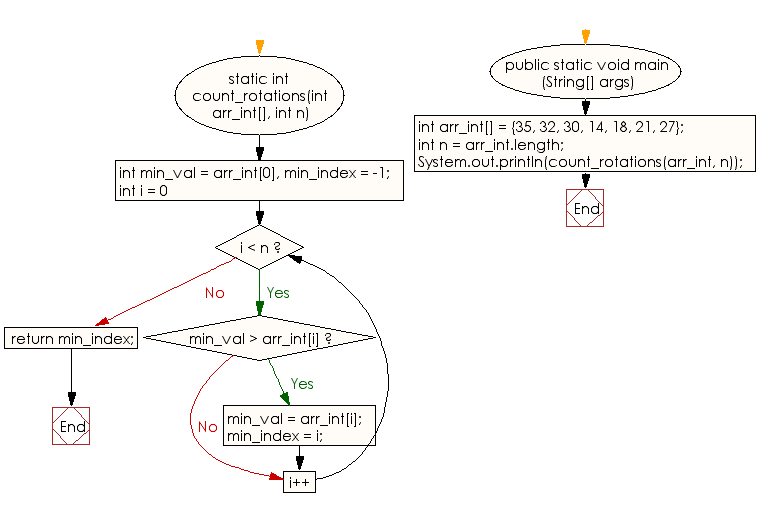﻿ Java: Find the rotation count in a rotated sorted array# Java Array Exercises: Find the rotation count in a given rotated sorted array of integers

## Java Array: Exercise-47 with Solution

Write a Java program to find the rotation count in a given rotated sorted array of integers.

Sample Solution:

Java Code:

``````import java.util.*;
import java.lang.*;
import java.io.*;

public class Main
{
static int count_rotations(int arr_int[], int n)
{
int min_val = arr_int, min_index = -1;
for (int i = 0; i < n; i++)
{
if (min_val > arr_int[i])
{
min_val = arr_int[i];
min_index = i;
}
}
return min_index;
}
public static void main (String[] args)
{
int arr_int[] = {35, 32, 30, 14, 18, 21, 27};
// int arr_int[] = {35, 32, 14, 18, 21, 27};
// int arr_int[] = {35, 14, 18, 21, 27};
int n = arr_int.length;
System.out.println(count_rotations(arr_int, n));
}
}
```
```

Sample Output:

```
3
```

Flowchart:Visualize Java code execution (Python Tutor):

Java Code Editor:

Improve this sample solution and post your code through Disqus

What is the difficulty level of this exercise?

Test your Programming skills with w3resource's quiz.

﻿

## Java: Tips of the Day

countOccurrences

Counts the occurrences of a value in an array.

Use Arrays.stream().filter().count() to count total number of values that equals the specified value.

```public static long countOccurrences(int[] numbers, int value) {
return Arrays.stream(numbers)
.filter(number -> number == value)
.count();
}
```

Ref: https://bit.ly/3kCAgLb